[BACK]
 Computer Systems Science & EngineeringDOI:10.32604/csse.2023.029148Article

Effective Customer Review Analysis Using Combined Capsule Networks with Matrix Factorization Filtering

1Department of Computer Science and Engineering, Sree Sakthi Engineering College,Coimbatore, Tamilnadu, 641104, India
2Department of Information Technology, Thiagarajar College of Engineering, Madurai, Tamilnadu, 625015, India
3Department of Computer Science and Engineering, Sethu Institute of Technology, Virudhunagar, Tamilnadu, 626115, India
*Corresponding Author: K. Selvasheela. Email: selvaresearch2016@gmail.com
Received: 25 February 2022; Accepted: 01 April 2022

Abstract: Nowadays, commercial transactions and customer reviews are part of human life and various business applications. The technologies create a great impact on online user reviews and activities, affecting the business process. Customer reviews and ratings are more helpful to the new customer to purchase the product, but the fake reviews completely affect the business. The traditional systems consume maximum time and create complexity while analyzing a large volume of customer information. Therefore, in this work optimized recommendation system is developed for analyzing customer reviews with minimum complexity. Here, Amazon Product Kaggle dataset information is utilized for investigating the customer review. The collected information is analyzed and processed by batch normalized capsule networks (NCN). The network explores the user reviews according to product details, time, price purchasing factors, etc., ensuring product quality and ratings. Then effective recommendation system is developed using a butterfly optimized matrix factorization filtering approach. Then the system’s efficiency is evaluated using the Rand Index, Dunn index, accuracy, and error rate.

Keywords: Recommendation system; customer reviews; amazon product kaggle dataset; batch normalized capsule networks; butterfly optimized matrix factorization filtering

1  Introduction

To reduce the bad reviews, fake feedbacks, and bad impressions, it has to be recognized instantly. With the development of techniques, 95% of people purchase the products according to user reviews, ratings, and feedback—the complexity in manual review analysis is avoided by creating the automatic review analysis system . The automatic system uses the opinion mining concepts because it includes several techniques like data mining, text mining, artificial intelligence, and machine learning . These techniques help to derive human thoughts, ideas, and patterns from the unstructured text. The opinion mining concept utilizes the rule and learning-based analysis that helps to maximize the customer review analysis and minimize the computation complexity . Here, emotion, fine-grained, aspect, and intent-based approaches effectively derive negative and positive comments successfully. However, the main problem of existing technologies is giving inadequate accuracy, failure to perform perfectly in different domains, insufficient labeled data affecting the system’s performance, and difficulties in complex sentence computations. In addition to this, the long sequence reviews are consuming more time and create complexities.

This work introduces batch normalized capsule networks with an optimized matrix factorization filtering approach to overcome these issues. The normalization technique effectively works on the collected data by reducing the overfitting issues. The neural network uses the learning patterns that resolve the unlable related data issues and maintains the truthfulness against the fake review.

Then the rest of the paper is manipulated as follows; Section 2 analyzes the various researcher’s analyses in customer review analysis. Section 3 evaluates the working process of batch normalized capsule networks with an optimized matrix factorization filtering approach based on review analysis. Section 4 discusses the efficiency of the introduced system and the conclusion derived in Section 5.

2  Related Works

YousefKilani et al.  recommended the genetic algorithm with neighborhood technique to develop the hybrid recommendation system. This system collects the data from CiaoDVD, FilmTrust, and MovieLens, processed by the neighborhood collaborative filtering approach. The method computes the latent factor for every item, and the most preferred products are grouped. The searching process is optimized by applying the genetic algorithm that improves the overall clustering rate with minimum computation complexity.

Katarya et al., 2016  developed the recommendation system using the particle swarm optimized collaborative filtering technique. This system aims to reduce the computation complexity by applying the division and classification method. Here, the K-means clustering algorithm is applied for similar grouping customers. The clustering process is further improved by applying the particle swam characteristics. This process helps identify the most relevant purchasing item of the user that is used to create the optimized recommendation system. Yi. et al., 2020  created the machine learning technique-based customer sentiment analysis system for improving business growth. The system is developed according to user experience, and the review information is gathered from unified computing systems. The gathered data is visualized by applying a machine learning technique that recognizes the customer reviews upto 96% accuracy with a 0.6 error rate. To create an effective recommendation system, Maleki Shoja et al., 2020  applied a deep neural network on customer reviews. This system aims to analyze the unstructured and huge volume of data with minimum complexity. Here, the data is collected from Amazon.com, which is processed by the Latent Dirichlet Allocation process. The method extracts the features from the review by eliminating the ambiguity, redundancy, and sparsity issues. The deep learning technique executes the extracted features, and the matrix factorization is applied to form the cluster. The successful utilization of latent factors improves the overall system’s accuracy.

Smetanin et al., 2019  analyze the Russian product reviews using convolution neural networks. This work creates the training set according to the top-ranked reviews and sentiment lexicons. The extracted features are processed by a multiple-layer convolute network that helps to predict the new reviews effectively. This system minimizes the deviation errors and improves the overall accuracy up to 75.45%. Hussain et al., 2020  recommend a linguistic and spammer-based behavior model to detect spam reviews. This system solves the spam behavior prediction problem and creates the attention of the researchers using the behavior model. The model extracts the features from the linguistic reviews that the behavior model processes. The method computes the user reviews up to 93.1% of accuracy compared to the existing models.

Zhang et al., 2020  developed the multiclassification model’s e-commerce sentiment review analysis system. This system uses the directed weight model that identifies the relationship between the vocabulary and attributes. According to the relation, a directed graph is created, which helps to identify the similarity between the nodes. The computed similarity values are more useful to predict the sentiment and expressions with good accuracy. According to the various researcher’s opinions, the customer review analyze is played a major business. Several machine learning techniques are applied to predict the user reviews because the fake review influences the business growth. Although, existing techniques fail to identify the truthfulness of review with minimum classification problems. Therefore, in this work, optimized techniques are applied to reduce the difficulties and discuss research issues. The detailed working process of the introduced system is defined below section.

3  Batch Normalized Capsule Networks Based Customer Review Analysis

The good and best reviews help to maximize the business progress and sales rate because peoples are interested in purchasing their product via the ratings. With techniques, customers have influenced the business and created a bad impression on a specific product. The automatic systems are failing to predict the review trustfulness due to the minimum training patterns. The length of the reviews also affects the quality of the review and creates complexity while predicting false reviews. Then the main intention of this study is to maximize the false review prediction accuracy with minimum computation efforts. Therefore, this work utilizes optimized techniques such as batch normalized capsule networks and butterfly optimized matrix factorization filtering approaches to create an effective recommendation system.

Materials and Methods

These three datasets are utilized to examine the introduced system efficiency as given in Tab. 1. The effectiveness is measured in how effectively the system resolves the maximum error-rate classification and computation complexity issues. This work applies batch normalized capsule networks with an optimized matrix factorization filtering approach to resolve the existing issues. Then the overall working process of customer review analysis structure is illustrated in Fig. 1.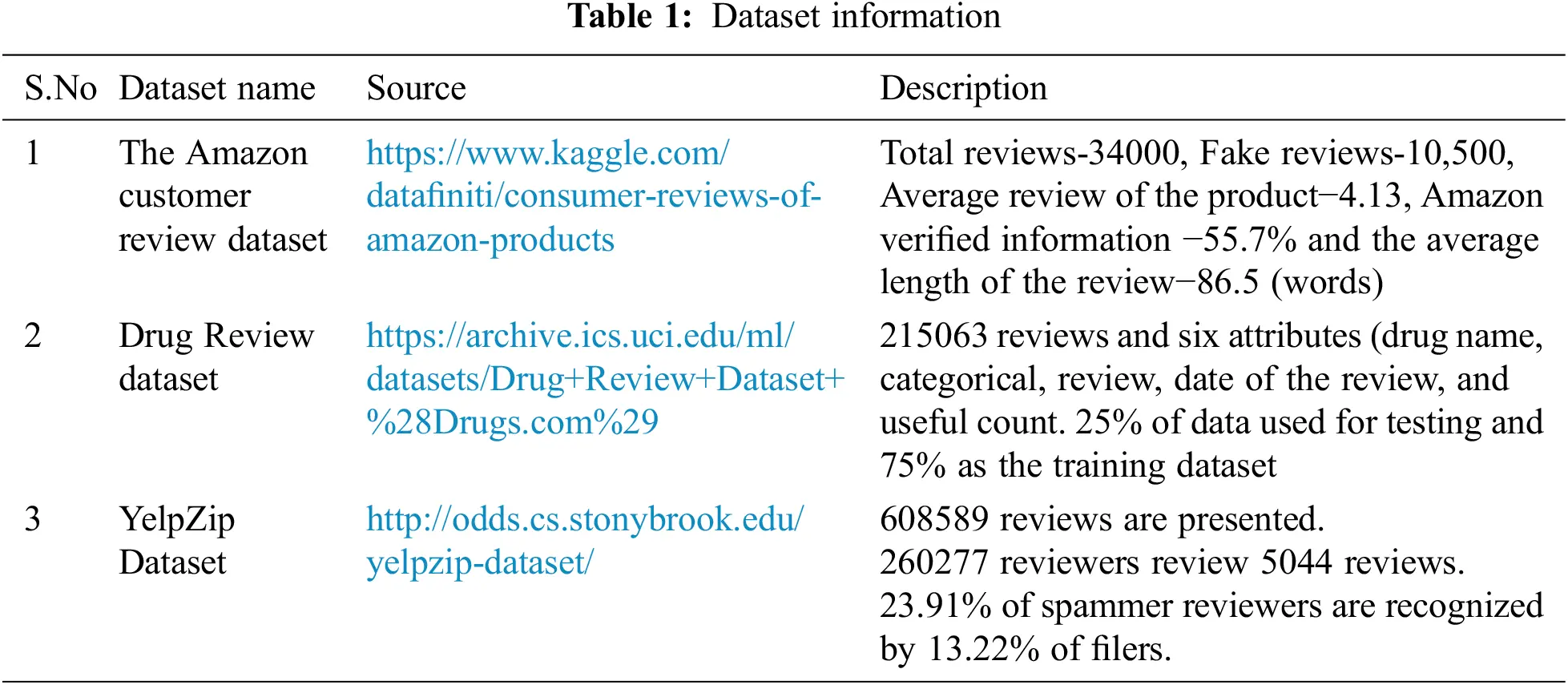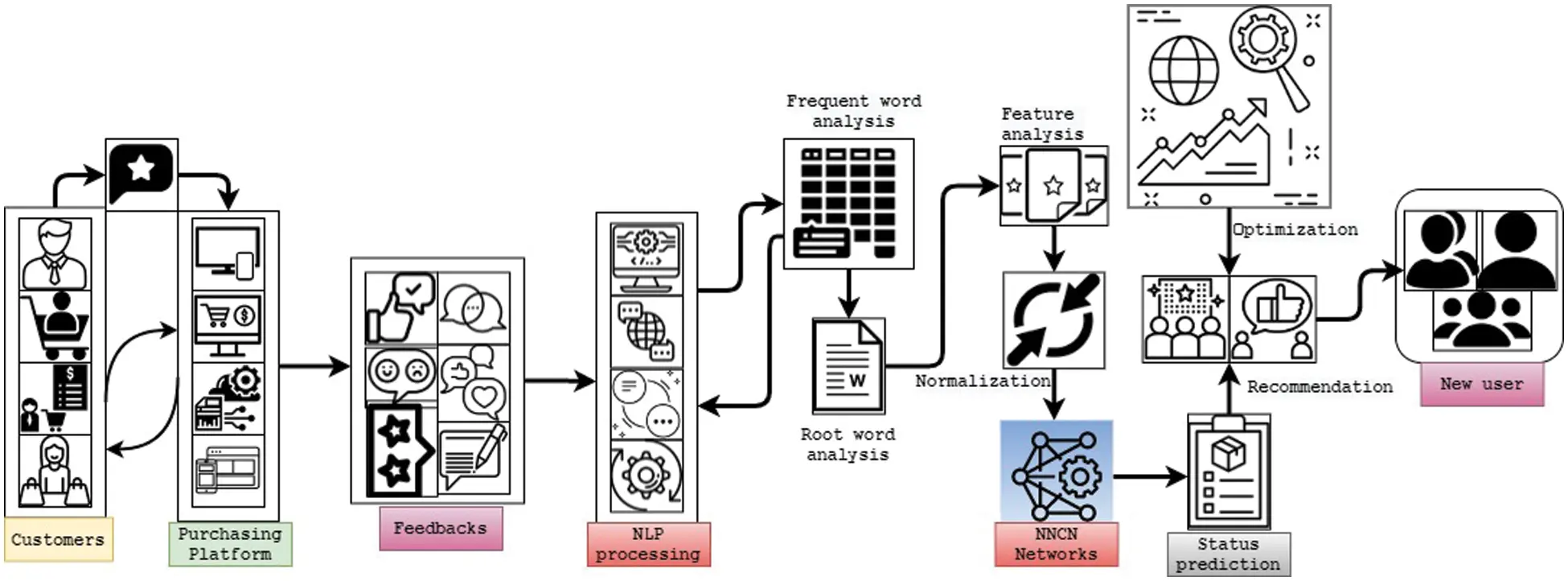Figure 1: Customer review analysis framework

The customer review analysis framework intends to minimize the computation complexity and maximize the computation speed. This is achieved by applying the batch norm process that normalizes the capsule network layers by re-scaling and re-centering the inputs. The normalization process resolves the internal covariate shift problem, i.e., network activation function distribution changes. This problem occurred because of the changes in network parameters during input training. The changes in learning parameters reduce by incorporating the butterfly optimization algorithm because it only smoothens the network objective function. The normalization is performed in the training set that fixes the inputs via computing variance and mean value. The normalization is done as mini-batch(B) processing.

Let, (B) is the size of m applied on the training set; for normalizing mean μB and variance σB2 is calculated.

μB=1mi1mxi (1)

σB2=1mi=1m(xiμB)2 (1a)

The computed (1) and (1a) is calculated for every layer l with d dimensional inputs x = (x(1), x(2), …x(d)). Therefore, the normalization (re-scaling and re-centered) should perform on each input individually, Eq. (1b).

x^i(k)=xi(k)μB(k)σB(k)2+ε (1b)

The re-centered and re-scaling of input x^i(k) is estimated from the mean μB(k) and variance σB(k)2 ; where i ∈ (1, m). This computation utilizes the ε small constant value to maintain the system stability. Suppose the calculation does not have ε value, then μB(k)=0 and σB(k)2=1 . Network power is restored after normalizing the network layer via the network learning process Eq. (2).

yi(k)=γ(k)x^i(k)+β(k) (2)

Here, the network output is calculated yi(k) via the mathematical operations of re-centered input x^i(k) with network parameters (γ(k) − weight, β(k) − bias). At last, the normalization process is represented in terms of BNγ(k),β(k):x1,,.m(k)y1,,.m(k) . For every input, x1,,.m(k) the output y1,,.m(k) is generated using the batch normalized network parameters. Then the computed yi(k) is transferred to the next layer yi(k)=BNγ(k),β(k)(x(k)) and the normalized output stays on the internal layer to the current layer. The batch normalization process has differentiable operations that reduce the gradient loss by using the chain rule. As said, the output is computed according to the activation function and gradient value. Hence, the derivate of the output lyi(k) is calculated concerning the choice of the activation function.

lx^i(k)=lyi(k)γ(k)lγ(k)=i=mlyi(k)x^i(k)lβ(k)=i=mlyi(k)} (3)

As said, the derivative of the normalized value should compute when optimizing the network parameters Eq. (3a)

lσB(k)2=i=1mlyi(k)(xi(k)μB(k))(γ(k)2(σB(k)2+ϵ)32)lμi(k)=i=1mlyi(k)γ(k)σB(k)2+ϵ+lσB(k)21mi=1m(2)(xi(k)μB(k))} (3a)

Here, the re-center and re-shaped values are obtained from the derivation of mean lμB(k) and variance lσB(k)2 . The variance value is calculated from the deviation between the input value xi(k) and mean value μB(k) . This computation uses the small constant ε along with the σB(k)2 and network parameters γ(k). The calculation helps to optimize the network process and improves the overall review analysis process. Similarly, the mean derivative value is estimated by analyzing the network parameter γ(k), deviation σB(k)2 , small constant ε and (xi(k)μB(k)) values. The input is processed in every input layer; therefore, input derivation values are computed using Eq. (3b).

lxi(k)=lx^i(k) 1σB(k)2+ϵ+lσB(k)22 (xi(k)μB(k))m+lμB(k)1m (3b)

The training process backpropagates the values for every normalization process. Here, the normalized value derivative lx^i(k)  is used to get the optimized input values lxi(k) . The lx^i(k)  value is computed from Eq. (3) with network parameters lyi(k)γ(k) . Then, mean μB(k) and variance σB(k)2 derivatives are utilized with m size mini-batch (B) normalization. The batch normalization in the capsule network process is illustrated in Fig. 2.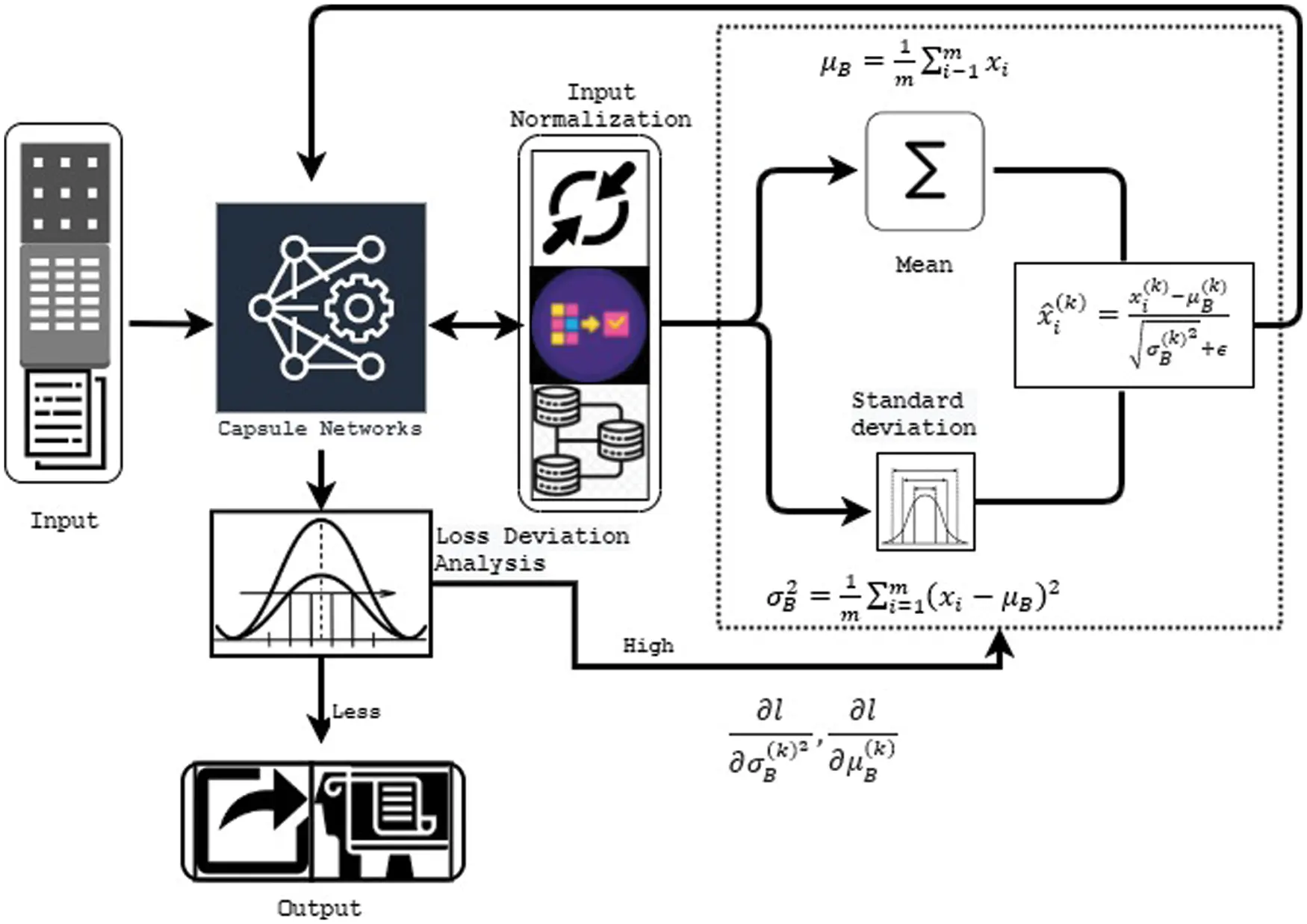Figure 2: Batch normalization in capsule network framework

The capsule network examines the incoming inputs for classifying the customer review status. The reviews are generally in the text format; it has been converted into the matrix format to be treated as an image, in the generated matrix, row associated with word or token. The created matrix is 28 * 28, fed into the convolution layer, which investigates the input with 256 kernels. The output is analyzed in the ReLu activation layer of 9*9 size of 2 strides. Finally, the feature map tensor is computed with 6 * 6 * 256 for a single capsule in layer l. Hence the network uses around 66(2568)=1152lowerlevelofcapsule . The computed output is fed into the normalization process defined in the above section. Here, mean μB(k) and variance σB(k)2 Values are utilized to estimate the output in the learning process. For every capsule in layer l bias value is β(k) is zero, and the output is estimated by using the softmax activation function (δ). The computed value is updated in every capsule ci ← δ(β(k)). The output of li is transferred to next layer lj (l + 1), and the output is estimated as sii cijμj|i) . Then capsule of the j layer should be computed as vjsquash(si) . Therefore, the entire output is estimated as βij(k)βij(k)+μj|i.vj . Once the loss deviations are very low, the computed output is compared with the training set, and respective outputs are derived. If the loss deviations are high, then the input derivatives are computed to re-calculate the input. According to the output, the review status is predicted, and the customer ratings are further examined by matrix factorization filtering to create the recommendation system. The method examines the inputs and is divided into two lower-dimensional matrices to reduce the computation complexity. The formed results are more useful to improve the product popularity identification and user preference. Here, user reviews are investigated for generating the matrix according to user ratings. The matrix is generated in terms of user and their items. The link between the user and their purchased items is referred to as the latent factor. Therefore, ratings between the user and the items are computed using Eq. (4a)

R^=HWR^RusersitemsHRuserslatentfactorWRlatentfactoritems} (4a)

The overall prediction rating R^=HW is computed for usersitems which is concerned in terms of userslatent factor and latent factoritems. After that, the user purchased item rating is calculated as (4b)

rui=f=0nfactorsHu,fWf,i (4b)

Here, the latent factor is estimated from the frequent or top reviewed product, which helps to improve the recommendation quality. As said here, the overfitting issues are resolved by applying the normalization process. During the user and items related preference identification process, the searching is improved by applying optimization techniques. This is achieved by applying the butterfly optimization algorithm. The algorithm works according to the fragrance fr and stimulus intensity (SI). These characteristics are utilized to select the objective function and network parameters (γ(k) − weight, β(k) − bias) updated. The fragrance value of each network parameter is estimated using Eq. (5a)

fr=smSIe (5a)

These parameters are calculated using sensory modality sm and exponent modality e. The computed values are from 0 to 1. After that, network parameters are searched locally and globally.

buit+1=buit+(rnd2hbuit)fru (5b)

For every iteration, the global solution is gotten from bu the butterfly moves, and the current best solution is identified as Eq. (5c)

buit+1=buit+(rnd2bvitbwit)fru (5c)

The Eq. (5c) is indicated as the local search part of the BOA. Where bvit and bwit are v-th and w-th butterflies from solution space. If bvit and bwit Butterflies belong to the same swarm, and rnd is a random number between the range [0,1] then the Eq. (5c) becomes a local random walk. Based on these procedures, the network parameters are updated continuously. The working process of the capsule network and the recommending process is illustrated in Fig. 3.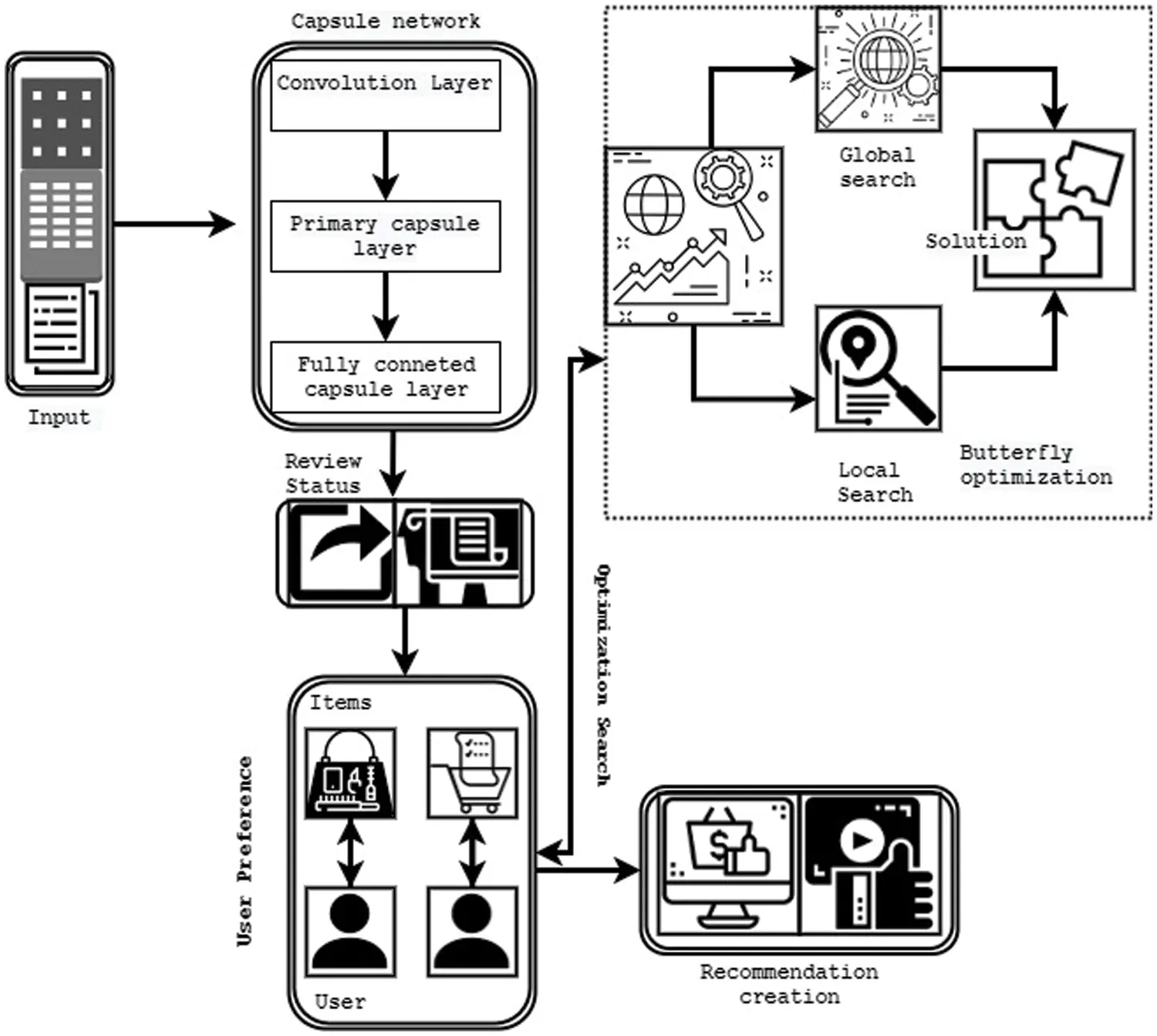Figure 3: Recommendation system framework

Then the recommending problem is overcome by minimizing the matrix factorization objective function defined in Eq. (6).

argminH,WRR^F+αH+βW (6)

Here, the network errors such as maximum error-rate classification problem, recommending problem, and stability issues are resolved by applying the optimized intelligent techniques. Further, the network overfitting problem is overcome using natural language processing approaches. These approaches have fine-tuned the inputs that maximize the review analysis accuracy. The user feedbacks are represented in many formats such as text, ratings, audio, and video. The original format consumes high computation complexity and time. Therefore, the unwanted information should be removed by performing token splitting, inflection removal, and stemming process. Initially, unwanted symbols like “,?,!” were removed from the feedback, which reviewed the status analysis process. Then reviews are checked in terms of URL because most of the fake reviews come from this category.

This root word is derived from the comment, which reduces the computation complexity and overfitting issues. The PoS() function is applied to the comment to get the parts of speech such as singular, adjective, verb, adverb, noun, and plural. Afterward appearance of words in the comment should examine to improve the review status analysis. The term frequency is computed using Eq. (7).

tf(woi,rej)=numberoftimeswoioccursinthesearchqueryrejtotalnumberofwordsinrej (7)

In Eq. (7), the word appeared the number of times is denoted as woi in the review rej and the entire amount of word presented in the review is represented as tf(woi, rej). The term frequency is further formulated using log normalization, which is performed using Eq. (8)

tf(te,r)=1+logfte,r (8)

In Eq. (8), te is the frequency of text appearing in review r.

After, the inverse document frequency of the word is estimated to identify the word is appears in repeated mode or rare mode in the review. The IDF is estimated as follows.

idf(r,D)=log|D|{rD:teD} (9)

In Eq. (9), |D| is the total number of reviews in the dataset {r ∈ D:te ∈ D} is represented as the number (count) of reviews that contains the word te. If the estimated IDF value is greater than 0, the TF-IDF is formed as using Eq. (10).

tfidf(te,r,D)=tf(te,r)idf(r,D) (10)

According to this computation, rarely appeared word is identified from the inverse document frequency value. If the word appeared in every review, it has 0 value else 1. The frequent appeared words are relevant to positive review and abnormal or rarely appeared words are negative. The computed information is given as input to the optimized capsule network that predict the review status effectively. Thus, the process reduces the overfitting issues successfully. The introduced system resolves the noise-related difficulties, normalization-based difficulties, and maximum error-rate classification problems completely. The effectiveness of the system evaluated using experimental results and analysis.

4  Results and Discussions

The effectiveness of the batch normalized capsule networks with optimized matrix factorization filtering approach (BNCN-OMF)-based customer review analysis is illustrated in this section. Here, three datasets such as Kaggle dataset, Drug review, and Yelpzip dataset information are utilized to investigating the introduced BNCN-OMF system excellency. The gathered reviews are analyzed by applying Natural language Processing toolkit that extracts the frequent of words and root words. The collected data is split into training (80%) and testing (20%) dataset which improves the overall review analysis. Initially, the batch normalization process applied on every layer in the capsule network which helps to reduce the overfitting and internal covariate shift problem. The continuous prediction of network parameter derivatives helps to minimize the issue and improve the prediction rate. After that, user-item relation and preference is computed to create the recommendation system. Here, butterfly optimization algorithm characteristics and functionalities improve the overall accuracy. The successful utilization of network parameter, normalization procedure and updating process minimize the deviation and improve the overall system’s performance. The maximum error-rate classification issue is examined using error rate metrics which are computed as follows.

MeanSquareErrorrate(MSER)=1Ni=1N(yiy^i)2 (11)

In Eq. (11), n is a total review in the dataset, yt is estimated output and y^ and predicted output. The cross-entropy value is calculated using Eq. (12).

Crossentropyloss(CEL)=c=1Myo,clog(po,c) (12)

In Eq. (12), M denoted as a review of the product

O-the observed value of the specific review,

P-observation of o, probability value during the prediction. Then the likelihood loss value is computed as follows.

likelihoodloss(LL)=1ni=1nlog(y^(i)) (13)

In Eq. (13), n-number of class, y is output. The introduced system effectiveness is evaluated using three dataset and the obtained results are illustrated in Tab. 2. The obtained results are compared with the existing researcher’s work such as [17,18,19] and  are compared with the introduced technique called the batch normalization deep learning network with ABC collaborative recommendation system (BNDNN-ABCRS)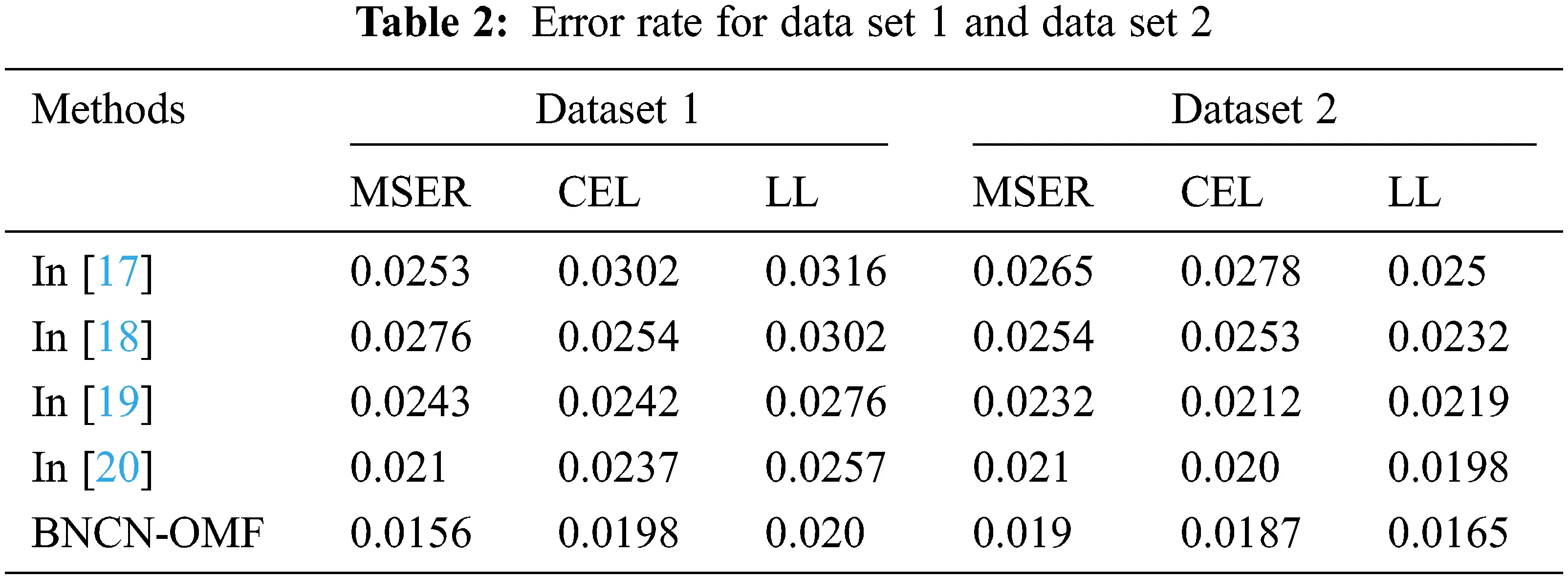Tabs. 2 and 3 illustrated that the error rate value of batch normalized capsule networks with optimized matrix factorization filtering approach (BNCN-OMF) based customer review analysis. Here, the method uses the normalization-based network parameter updating process. The normalization process computes the mean and standard deviation value for every inputs. The successive computation of these parameters x^i(k)=xi(k)μB(k)σB(k)2+ϵ reduces the deviation between the actual and computed output value. The minimum error value directly indicates that the maximum recognition accuracy also reduces the maximum error-rate classification problem effectively. The graphical evaluation is illustrated in Fig. 4.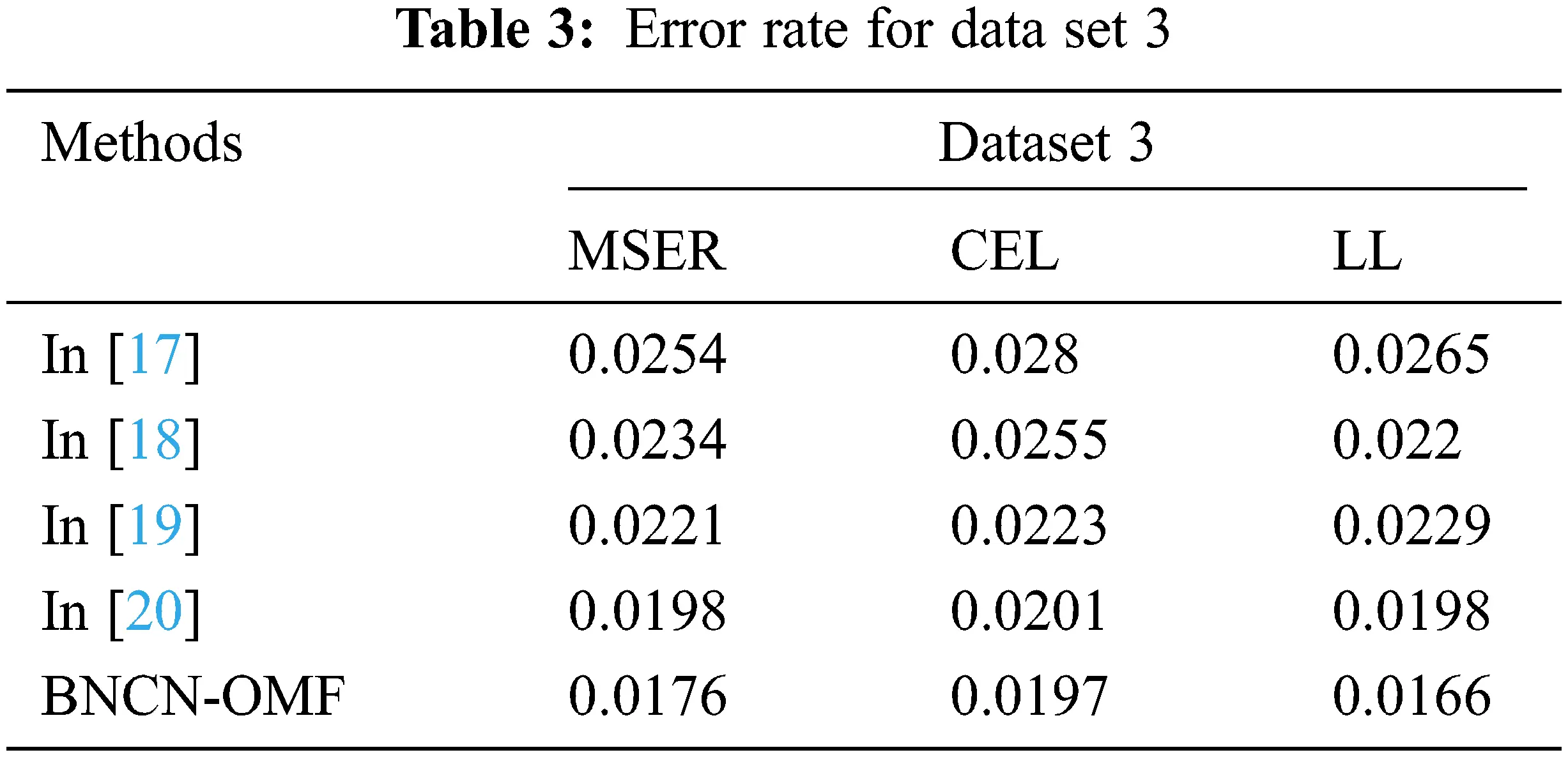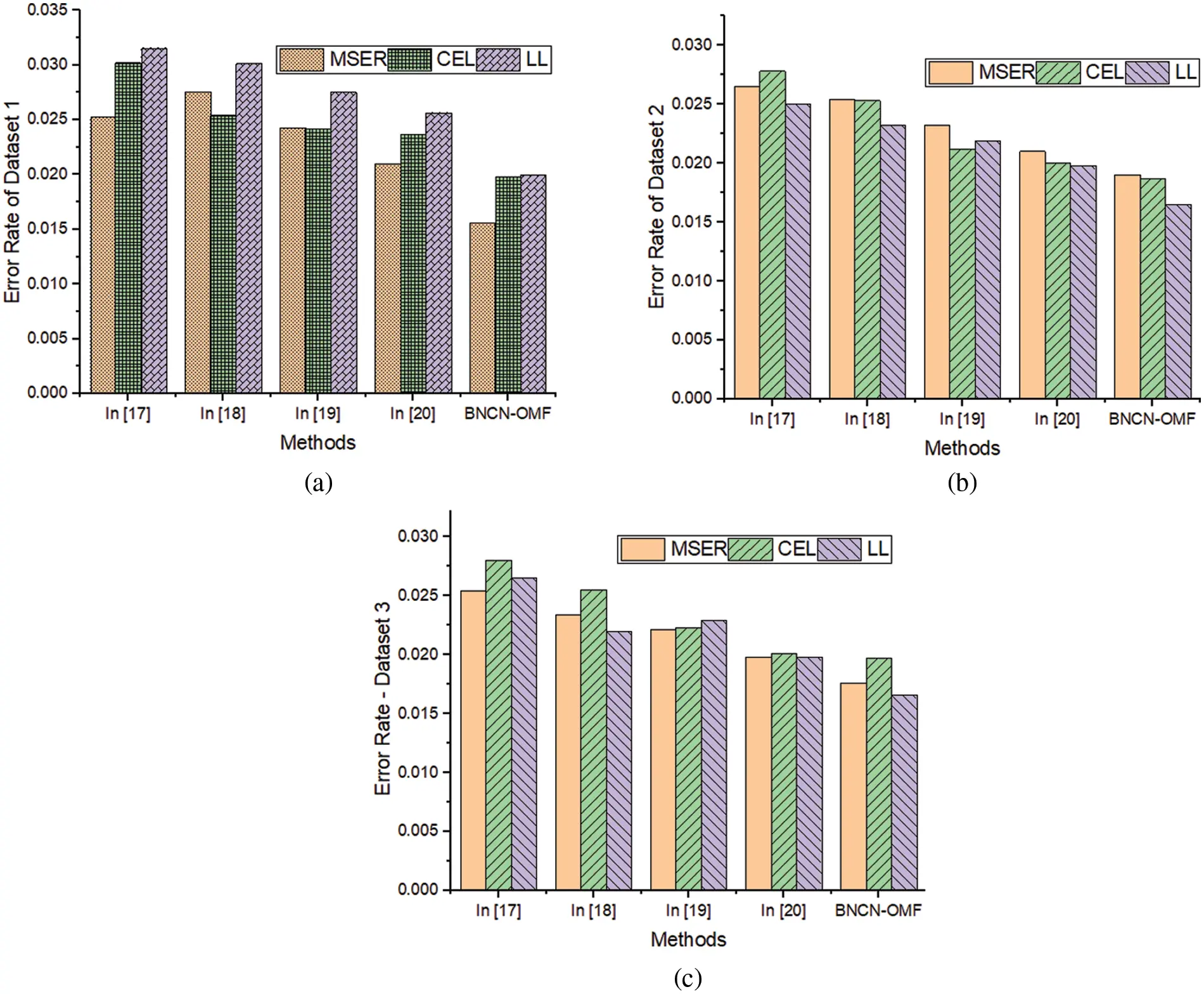Figure 4: Error rate value of various datasets a) Amazon customer review dataset, b) Drug review dataset and c) Yelpzip database

Fig. 4 illustrated that the batch normalized capsule networks with optimized matrix factorization filtering approach (BNCN-OMF) based customer review analysis. The method attains the minimum error rate while classifying the truth and fake reviews from the various datasets. According to the figure, BNCN-OMF approach has minimum error rate on three datasets ((dataset 1- MSER-0.0156, CEL-0.0198 and LL-0.02), (dataset 2- MSER-0.019, CEL-0.0187 and LL-0.0165), and (dataset 3- MSER-0.0176, CEL-0.0197 and LL-0.0166)). The obtained results are very low compared to the existing works. The BNCN-OMF approach uses the re-centered and re-scaled x^i(k)=xi(k)μB(k)σB(k)2+ϵ information using μB=1mi1mxi and σB2=1mi=1m(xiμB)2 which helps to normalize the input values. The effective utilization of small constant ε helps to predict the output yi(k)=γ(k)x^i(k)+β(k) with minimum deviation error. Here, the derivatives lx^i(k) 1σB(k)2+ϵ+lσB(k)22 (xi(k)μB(k))m+lμB(k)1m are utilized to reduce the maximum error-rate classification problem effectively. The minimum error rate directly indicates that the system ensures high recognition accuracy, and the obtained results are illustrated in Fig. 5.Figure 5: Dataset 1 (a) Sensitivity and (b) Specificity; dataset-2 (c) Sensitivity and (d) Specificity; dataset-3 (e) Sensitivity and (f) Specificity

Fig. 5 illustrated that sensitivity and specificity value of the batch normalized capsule networks with optimized matrix factorization filtering approach (BNCN-OMF). The method investigates the new customer review according to the previously trained information with high accuracy value. As said, the network parameters are updated according to the derivative values of the normalization parameters. This process reduces the error rate. During the classification process, capsule network uses the effective kernel value and weight updating procedure that causes to improve the overall recognition rate. The effectiveness of the system evaluated on three datasets and the system obtained the high sensitivity value and specificity value on all datasets. The obtained results are high compared to the other normal methods. Here, every layer computes the output by using the activation function that has been evaluated for every time. The computed value is updated in every capsule ci ← δ(β(k)) and transferred to next layer lj (l+1) and the output is estimated as sii cijμj|i) . Then capsule of j layer should be computed as vjsquash (si) . Therefore, the entire output is estimated as βij(k)βij(k)+μj|i.vj . the effective updating process and output computation procedure improve the review status identification rate. Then the obtained results are illustrated in Fig. 6.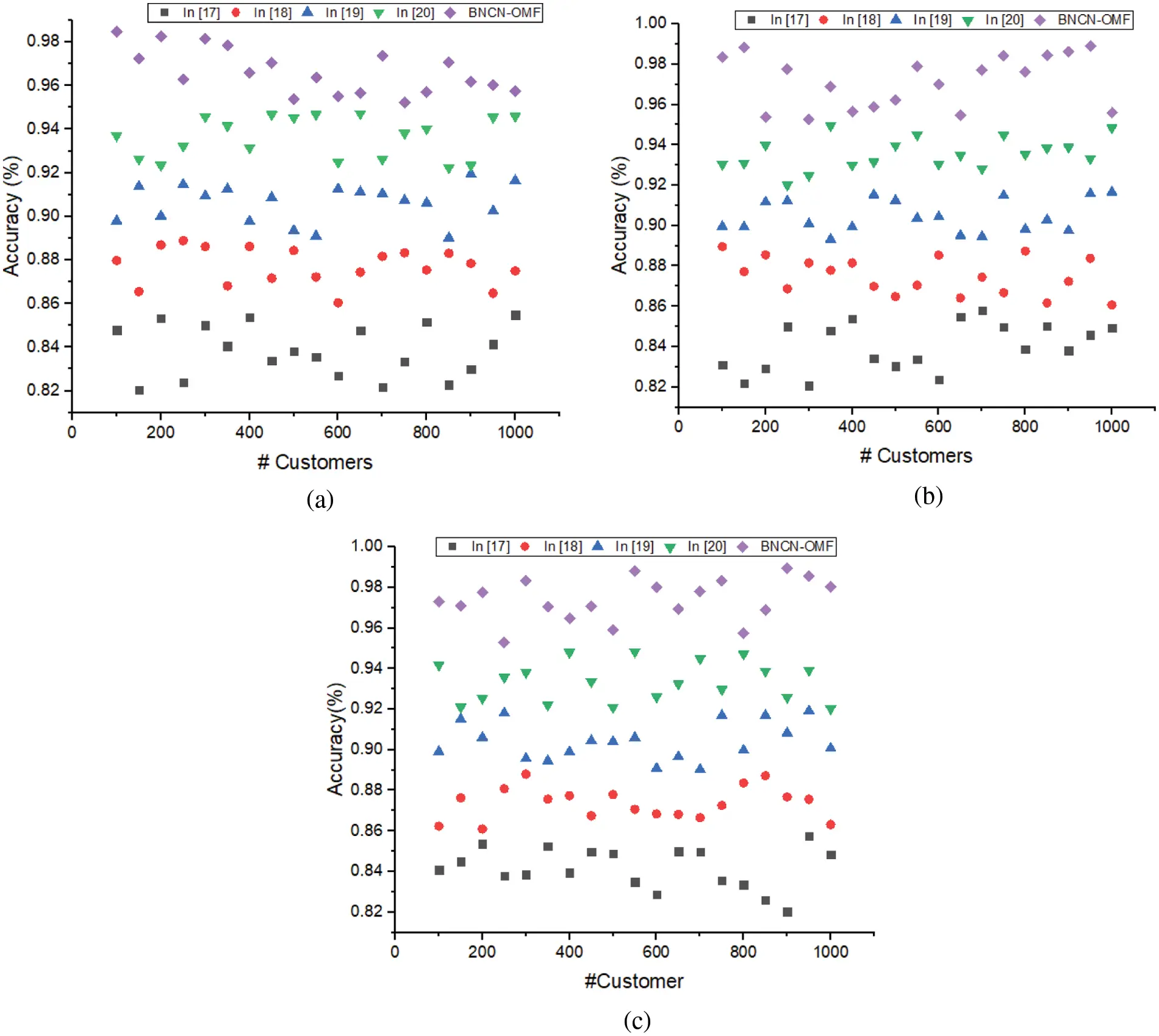Figure 6: (a) Dataset 1-accuracy (b)- Dataset-2-accuracy and (c) Dataset-3-accuracy

The above Fig. 6 illustrated that the accuracy of batch normalized capsule networks with optimized matrix factorization filtering approach (BNCN-OMF) based customer review analysis system. The method computes the output in every layer by normalizing the inputs using the derivatives of the mean and standard deviation value. This process minimizes the overfitting issue and error-rate problem. Further this process improves the network fine-tuning process by minimizing internal covariate shift problem. The successive utilization of network parameters causes to improve the overall recognition accuracy (fake and truth review) on all three datasets such as Kaggle dataset (98.9%), Drug review (99.27%), and Yelpzip dataset (98.92%). The obtained results are high compared to the existing approach. The effective recognition process helps to improve the overall recommendation system accuracy. The proficiency of the recommendation system evaluated using rand index (RI) and Dunn index.

5  Conclusion

Thus the paper analyzing the batch normalized capsule networks with optimized matrix factorization filtering approach (BNCN-OMF) based customer review analysis system. This system gathers the customer review from different datasets which processed by different procedures. Initially, the tokens are removed by applying the NLP tool and frequent word; root words are computed. Then, the identified features are investigated using the layer of capsule networks with respective kernel values. Every layer in the network normalized according to the mean and standard deviation derivative values. This process minimizes the overfitting problems while analyzing the input reviews. The continuous analysis, network parameter updating, backpropagating and normalization process classifies the fake and normal reviews. From that, user item preference is examined and generated effective recommendation system. The performance of this searching is improved by butterfly characteristics. The created system achieves the 99.27% accuracy by reducing the maximum error-rate classification problem. The optimized feature selection process is applied in future to improve the overall efficiency and reliability of the system.

Funding Statement: The authors received no specific funding for this study.

Conflicts of Interest: The authors declare that they have no conflicts of interest to report regarding the present study.

Reference

1. D. Chehal, G. Parul and G. Payal, “Implementation and comparison of topic modeling techniques based on user reviews in e-commerce recommendations,” Journal of Ambient Intelligence and Humanized Computing, vol. 5, no. 12, pp. 5055–5070, 202
2. L. Yang, L. Ying and W. Wang, “Sentiment analysis for e-commerce product reviews on sentiment lexicon and deep learning,” IEEE Access, vol. 8, no. 1, pp. 23522–23530, 2020.
3. A. Brahma and D. Rajasi, “Role of social media and e-commerce for business entrepreneurship,” IEEE Access, vol. 6, no. 6, pp. 1–18, 2020.
4. H. Syed Far Abid, X. Zhao and N. Mohammad, “Ubiquitous role of social networking in driving m-commerce: Evaluating the use of mobile phones for online shopping and payment in the context of trust,” SAGE Open, vol. 10, no. 3, pp. 213–221, 2020.
5. H. Peng, C. Erik and H. Amir, “A review of sentiment analysis research in Chinese language,” Cognitive Computation, vol. 9, no. 4, pp. 423–435, 2017.
6. H. Ahmed, T. Issa and S. Sherif, “Detection of online fake news using n-gram analysis and machine learning techniques,” in Int. Conf. on Intelligent, Secure, and Dependable Systems in Distributed and Cloud Environments, pp. 127–138. Springer, Cham, 2017.
7. X. Liu, S. Hyunju and C. Alvin, “Examining the impact of luxury brand’s social media marketing on customer engagement​: Using big data analytics and natural language processing,” Journal of Business Research, vol. 125, no. 10, pp. 815–826, 2019.
8. D. Cirqueira, P. Márcia and B. Thaís Braga, “Improving relationship management in universities with sentiment analysis and topic modeling of social media channels: Learnings from UFPA,” in Proc. of the Int. Conf. on web Intelligence, Leipzig Germany, pp. 998–1005, 2017.
9. M. Reisenbichler and R. Thomas, “Topic modeling in marketing: Recent advances and research opportunities,” Journal of Business Economics, vol. 89, no. 34, pp. 327–356, 201
10. B. Jeong, Y. Janghyeok and L. JaeMin, “Social media mining for product planning: A product opportunity mining approach based on topic modeling and sentiment analysis,” International Journal of Information Management, vol. 48, no. 5, pp. 280–290, 2019.
11. A. Amado, C. Paulo and R. Paulo, “Research trends on big data in marketing: A text mining and topic modeling based literature analysis,” European Research on Management and Business Economics, vol. 24, no. 1, pp. 1–7, 2018.
12. D. Patel, K. Aishwerya and S. Sameet, “Fake review detection using opinion mining,” International Research Journal of Engineering and Technology (IRJET), vol. 5, no. 1, pp. 192–201, 2018.
13. Y. Wu and N. Eric, “Fake online reviews: Literature review, synthesis, and directions for future research,” Decision Support Systems, vol. 132, no. 8, pp. 113280–113289, 2020.
14. J. Lei, G. Yaosong Guo and X. Zhongyuan, “Automatic generation system of distribution network switch test scheme based on topology analysis,” Journal of Physics: Conference Series, vol. 2005, no. 1, pp. 012221–012226, 2021.
15. F. Hemmatian and S. Mohammad Karim, “A survey on classification techniques for opinion mining and sentiment analysis,” Artificial Intelligence Review, vol. 52, no. 3, pp. 1495–1545, 2019.
16. H. C. Soong, N. B. Jalil and R. Kumar Ayyasamy, “The essential of sentiment analysis and opinion mining in social media : Introduction and survey of the recent approaches and techniques,” IEEE 9th Symposium on Computer Applications & Industrial Electronics (ISCAIE), Malaysia, pp. 112–119, 2019.
17. A. YousefKilani, A. FawziOtoom and A. AlsarhanManal, “A genetic algorithms-based hybrid recommender system of matrix factorization and neighborhood-based techniques,” Journal of Computational Science, vol. 28, no. 1, pp. 78–93, 20
18. R. Katarya and O. P. Verma, “A collaborative recommender system enhanced with particle swarm optimization technique,” Multimed Tools Appl, vol. 75, no. 5, pp. 9225–9239, 2016.
19. S. Yi and X. Liu, “Machine learning based customer sentiment analysis for recommending shoppers, shops based on customers’ review,” Complex Intellegence. System, vol. 6, no. 5, pp. 621–634, 2020.
20. B. Maleki Shoja and N. Tabrizi, “Customer reviews analysis with deep neural networks for e-commerce recommender systems,” IEEE Access, vol. 7, no. 5, pp. 119121–119130, 2019.
21. S. Smetanin and M. Komarov, “Sentiment analysis of product reviews in Russian using convolutional neural networks,” 2019 IEEE 21st Conference on Business Informatics (CBI), pp. 482–486, 2019.
22. N. Hussain, H. Turab Mirza and I. Hussain, “Spam review detection using the linguistic and spammer behavioral methods,” IEEE Access, vol. 8, no. 5, pp. 53801–53816, 2020.
23. S. Zhang, D. Zhang, H. Zhong and G. Wang, “A multiclassification model of sentiment for e-commerce reviews,” IEEE Access, vol. 8, no. 5, pp. 189513–189526, 2020.This work is licensed under a Creative Commons Attribution 4.0 International License, which permits unrestricted use, distribution, and reproduction in any medium, provided the original work is properly cited.